Courses

# RD Sharma Solutions -Ex-23.1 (Part - 1), Graphical Representation Of Statistical Data, Class 9, Math Class 9 Notes | EduRev

## Class 9: RD Sharma Solutions -Ex-23.1 (Part - 1), Graphical Representation Of Statistical Data, Class 9, Math Class 9 Notes | EduRev

The document RD Sharma Solutions -Ex-23.1 (Part - 1), Graphical Representation Of Statistical Data, Class 9, Math Class 9 Notes | EduRev is a part of the Class 9 Course RD Sharma Solutions for Class 9 Mathematics.
All you need of Class 9 at this link: Class 9

Q1: The following table shows the daily production of T.V. sets in an industry for 7 days of a week.

Represent the above information by a pictograph.

 Day Monday Tuesday Wednesday Thursday Friday Saturday Sunday Number of tv sets 300 400 150 250 100 350 200

The given information can be represented using a pictograph in the following manner: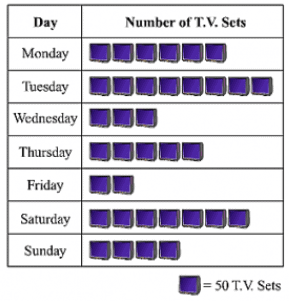Q2: The following table shows the number of Maruti cars sold by five dealers in a particular month:

Represent the above information by a pictograph.

 Dealer saya Bagga links D.D Motors Bhasin Motors Competent Cars sold 60 40 20 15 10

The given information can be represented using a pictograph in the following manner: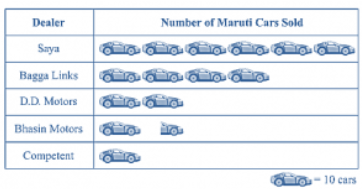Q 3: The population of Delhi State in different census years is as given below:

 Census year 1961 1971 1981 1991 2001 Population in Lakhs 30 55 70 110 150

Represent the above information with the help of a bar graph.

While drawing a bar graph, we keep in mind that:

1. The width of the bars should be uniform throughout.
2. The gap between any two bars should be uniform throughout.
3. Bars may be either horizontal or vertical.

To represent the given data by a vertical bar graph, we first draw horizontal and vertical axes.

Let us consider that the horizontal and vertical axes represent the years and the population in lakhs respectively. We have to draw 5 bars of different heights given in the table. At first, we mark 5 points in the horizontal axis at equal distances and erect rectangles of the same width at these points. The heights of the rectangles are proportional to the population in lakhs. The vertical bar graph of the given data is following: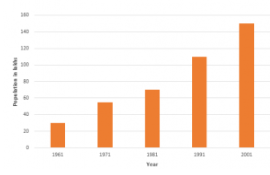Note that each bar is of the same width and the gap between them is uniform. Make sure that the width of the bars and the gap between them should not be necessarily same.

Q4: Read the bar graph shown in the figure and answer the following questions:

(i) What is the information given by the bar graph?

(ii) How many tickets of Assam State Lottery were sold by the agent?

(iii) Of which state, were the maximum number of tickets sold?

(iv) State whether true or false.

The maximum number of tickets sold is three times the minimum number of tickets sold.

(v) Of which state were the minimum numbers of tickets sold?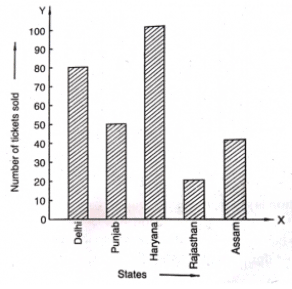Ans:

(1) The bar graph represents the number of tickets of different state lotteries sold by an agent on a day.

(2) The number of tickets of Assam State Lottery were sold by the agent is 40.

(3) The maximum numbers of tickets were sold is 100, in the state Haryana

(4) The maximum number of tickets were sold is 100, in the state Haryana the minimum number of tickets were sold is 20, in the state Rajasthan. It is clear that 100 are equal to the 5 times of 20. Hence, the statement is false.

(5) The minimum numbers of tickets were sold is 20, in the state Rajasthan.

Q5: Study the bar graph representing the number of persons in various age groups in a town shown in figure Observe the bar graph and answer the following questions:

(i) What is the percentage of the youngest age-group persons over those in the oldest age group?

(ii) What is the total population of the town?

(iii) What is the number of persons in the age-group 60-65?

(iv) How many persons are more in the age-group 10-15 than in the age group 30-35?

(v) What is the age-group of exactly 1200 persons living in the town?

(vi) What is the total number of persons living in the town in the age-group 50-55?

(vii) What is the total number of persons living in the town in the age-groups 10-15 and 60-65?

(vii) Whether the population in general increases, decreases or remains constant with the increase in the age-group.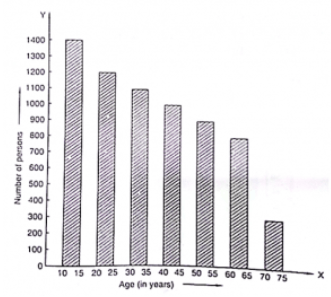Ans:

(1) The youngest age-group is 10-15 years. The number of persons belonging to this group is 1400. The oldest age-group is 70-75 years. The number of persons belonging to this group is 300. The percentage of youngest age-group persons over those in the oldest group is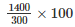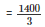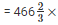(2) The population of the town is 300+ 800+900+1000 +1100 +1200+1400 = 6700

(3) The number of persons in the age group 60 – 65 is 800.

(4) The number of persons in the age group 10 – 15 is 1400 and the number of persons in the age group 30-35 is 1100. Hence the number of more persons in the age group 10 – 15 than the group 30-35 is 1400 — 1100 = 300.

(5) The age group of 1200 persons living in the town is 20 – 25.

(6) The total number of persons living in the town in the age-group 50 – 55 is 900.

(7) The total number of persons living in the town in the age-groups 10 -15 and 60 – 65 is 1400 + 800 = 2200.

(8) It is shown from the bar graph that the height of the bars decreases as the age-group increases. Hence, the population decreases with the increases in the age-group.

Q6: Read the bar graph shown in the figure and answer the following questions: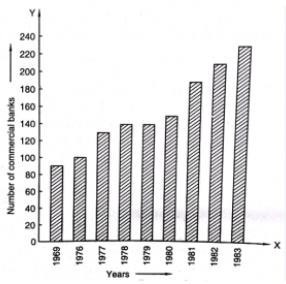(i) What is the information given by the bar graph?

(ii) What was the number of commercial banks in 1977?

(iii) What is the ratio of the number of commercial banks in 1969 to that in 1980?

(iv) State whether true or false:

The number of commercial banks in 1983 is less than double the number of commercial banks in 1969.

Ans:

(1) The bar graph represents the number of commercial banks in India during some particular years.

(2) The number of commercial banks in 1977 was

120 + (140 – 120)/2

⇒ = 120+ 20/ 2

⇒ = 120 + 10

⇒ 130

(3) The number of commercial banks in 1969 was

80 + (100 – 80)/ 2

⇒ = 80 + 20/2

⇒ = 80 + 10

⇒ = 90

The number of commercial banks in 1980 was

140 + (160 -140)/ 2

⇒ = 140 +20/ 2

⇒ =140+10

= 150

Hence, the required ratio is 90/150

⇒ 3/5

⇒ 3 : 5

(4) The number of commercial banks in 1983 was

220 + (240-220)/ 2

⇒  = 220 + 20/2

⇒ = 220 +10

⇒ = 230

The number of commercial banks in 1969 was 90.

When we multiply this number by 2, it becomes 2 x 90 =180

Clearly, 230 is not less than 180. Hence the statement is false.

Q7: Given below is the bar graph indicating the marks obtained out of 50 in mathematics paper by 100 students. Read the bar graph and answer the following questions.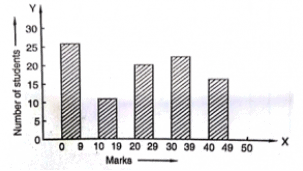i) It is decided to distribute workbooks on mathematics to the students obtaining less than 20 marks, giving one workbook to each of such students. If a workbook costs Rs. 5, what sum is required to buy the workbooks?

(ii) Every student belonging to the highest mark group is entitled to get a prize of Rs. 10. How much amount of money is required for distributing the prize money?

(iii) Every student belonging to the lowest mark-group has to solve 5 problems per day. How many problems, in all, will be solved by the students of this group per day?

(iv) State whether true or false.

(a) 17% students have obtained marks ranging from 40 to 49.

(b) 59 students have obtained marks ranging from 10 to 29.

(v) What is the number of students getting less than 20 marks?

(vi) What is the number of students getting more than 29 marks?

(vii) What is the number of students getting marks between 9 and 40?

(viii) What is the number of students belonging to the highest mark group?

(ix) What is the number of students obtaining more than 19 marks?

Ans :

(1) The number of students obtaining less than 20 marks is 27 + 12 = 39

Hence, the total cost to buy the work books is 5 x 39 = Rs. 195.

(2) The highest mark group is 40-49. The number of students belonging to this group is 17. Hence, the total amount of money required to distribute the prize money is 10 x 17 = Rs. 170

(3) The lowest mark group is 0-9. The number of students belonging to this group is 27. Hence, the total number of problems will be solved by the students of this group is 5 x 27 = 135

(4) The total number of students is 100 (given in the question.

(a) The number of students obtaining marks ranging from 40 – 49 is 17.

The percentage of students belonging to this group is (17/100) x100 = 17 %

Hence, the statement is true.

(b) The number of students obtaining marks ranging from 10 to 29 is 12 + 20 = 32

Hence, the statement is false.

(5) The number of students getting less than 20 marks is 27 + 12 = 39

(6) The number of students getting more than 29 marks is 24 + 17 = 41

(7) The number of students getting marks between 9 to 40 is 12 + 20 + 24 = 56

(8) The number of students belonging to the highest mark group 40 – 49 is 17.

(9) The number of students obtaining more than 19 marks is 20 + 24 + 17 = 61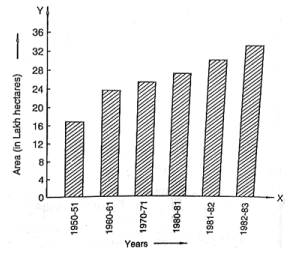(i) What is the information given by the bar graph?

(ii) State each of the following whether true or false.

(a) The number of government companies in 1957 is that of 1982 is 1 : 9.

(b) The number of government companies has decreased over the year 1957 to 1983.

Ans :

(1) The bar graph represents the number of government companies in India during some years.

(2) (a) The number of companies in 1957 was 50 and the number of companies in 1982 was 375. Their ratio in that order is 50: 375 = 2: 15

Hence, the statement is false.

(b) The height of the bar graphs increases over the years 1957 to 1983. Hence, the statement is false.

2:15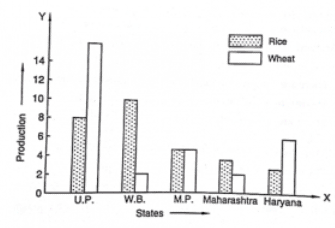(i) What information is given by the bar graph?

(ii) Which state is the largest producer of rice?

(iii) Which state is the largest producer of wheat?

(iv) Which state has total production of rice and wheat at its maximum?

(v) Which state has total production of wheat and rice at its minimum?

Ans:

(1) The bar graph represents the production of rice and wheat in different states of India.

(2) According to the height of the bars corresponding to rice, W.B. is the largest producer of rice.

(3) According to the height of the bars corresponding to wheat. U.P. is the largest producer of wheat.

(4) U.P. has the maximum total production of rice and wheat, which is 8 + 16 = 24 units

(5) Maharashtra has the minimum total production of rice and wheat, which are exactly 2 + 4 = 6 units.

The document RD Sharma Solutions -Ex-23.1 (Part - 1), Graphical Representation Of Statistical Data, Class 9, Math Class 9 Notes | EduRev is a part of the Class 9 Course RD Sharma Solutions for Class 9 Mathematics.
All you need of Class 9 at this link: Class 9Use Code STAYHOME200 and get INR 200 additional OFF Use Coupon Code
All Tests, Videos & Notes of Class 9: Class 9

### Top Courses for Class 9## RD Sharma Solutions for Class 9 Mathematics

91 docs

### Top Courses for Class 9Track your progress, build streaks, highlight & save important lessons and more!

,

,

,

,

,

,

,

,

,

,

,

,

,

,

,

,

,

,

,

,

,

,

,

,

,

,

,

,

,

,

;